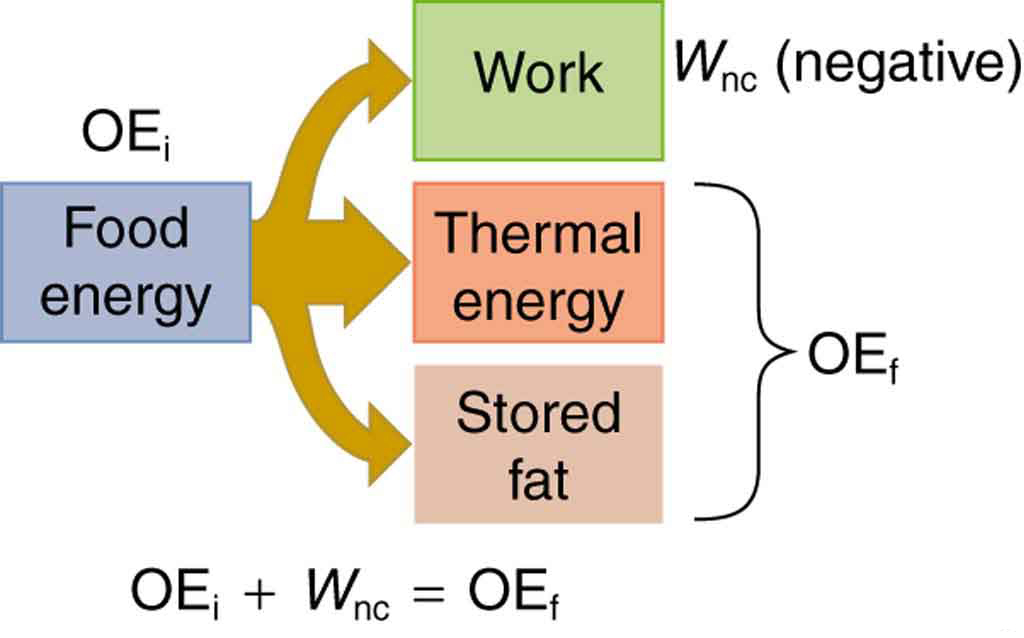# 7.8 Work, energy, and power in humans

 Page 1 / 7
• Explain the human body’s consumption of energy when at rest vs. when engaged in activities that do useful work.
• Calculate the conversion of chemical energy in food into useful work.

## Energy conversion in humans

Our own bodies, like all living organisms, are energy conversion machines. Conservation of energy implies that the chemical energy stored in food is converted into work, thermal energy, and/or stored as chemical energy in fatty tissue. (See [link] .) The fraction going into each form depends both on how much we eat and on our level of physical activity. If we eat more than is needed to do work and stay warm, the remainder goes into body fat.Energy consumed by humans is converted to work, thermal energy, and stored fat. By far the largest fraction goes to thermal energy, although the fraction varies depending on the type of physical activity.

## Power consumed at rest

The rate at which the body uses food energy to sustain life and to do different activities is called the metabolic rate    . The total energy conversion rate of a person at rest is called the basal metabolic rate    (BMR) and is divided among various systems in the body, as shown in [link] . The largest fraction goes to the liver and spleen, with the brain coming next. Of course, during vigorous exercise, the energy consumption of the skeletal muscles and heart increase markedly. About 75% of the calories burned in a day go into these basic functions. The BMR is a function of age, gender, total body weight, and amount of muscle mass (which burns more calories than body fat). Athletes have a greater BMR due to this last factor.

Basal metabolic rates (bmr)
Organ Power consumed at rest (W) Oxygen consumption (mL/min) Percent of BMR
Liver&spleen 23 67 27
Brain 16 47 19
Skeletal muscle 15 45 18
Kidney 9 26 10
Heart 6 17 7
Other 16 48 19
Totals 85 W 250 mL/min 100%

Energy consumption is directly proportional to oxygen consumption because the digestive process is basically one of oxidizing food. We can measure the energy people use during various activities by measuring their oxygen use. (See [link] .) Approximately 20 kJ of energy are produced for each liter of oxygen consumed, independent of the type of food. [link] shows energy and oxygen consumption rates (power expended) for a variety of activities.

## Power of doing useful work

Work done by a person is sometimes called useful work    , which is work done on the outside world , such as lifting weights. Useful work requires a force exerted through a distance on the outside world, and so it excludes internal work, such as that done by the heart when pumping blood. Useful work does include that done in climbing stairs or accelerating to a full run, because these are accomplished by exerting forces on the outside world. Forces exerted by the body are nonconservative, so that they can change the mechanical energy ( $\text{KE}+\text{PE}$ ) of the system worked upon, and this is often the goal. A baseball player throwing a ball, for example, increases both the ball’s kinetic and potential energy.

If a person needs more energy than they consume, such as when doing vigorous work, the body must draw upon the chemical energy stored in fat. So exercise can be helpful in losing fat. However, the amount of exercise needed to produce a loss in fat, or to burn off extra calories consumed that day, can be large, as [link] illustrates.

What is the difference between a principle and a law
the law is universally proved. The principal depends on certain conditions.
Dr
what does the speedometer of a car measure ?
Car speedometer measures the rate of change of distance per unit time.
Moses
describe how a Michelson interferometer can be used to measure the index of refraction of a gas (including air)
using the law of reflection explain how powder takes the shine off a person's nose. what is the name of the optical effect?
WILLIAM
is higher resolution of microscope using red or blue light?.explain
WILLIAM
can sound wave in air be polarized?
Unlike transverse waves such as electromagnetic waves, longitudinal waves such as sound waves cannot be polarized. ... Since sound waves vibrate along their direction of propagation, they cannot be polarized
Astronomy
A proton moves at 7.50×107m/s perpendicular to a magnetic field. The field causes the proton to travel in a circular path of radius 0.800 m. What is the field strength?
derived dimenionsal formula
what is the difference between mass and weight
assume that a boy was born when his father was eighteen years.if the boy is thirteen years old now, how is his father in
Isru
what is airflow
derivative of first differential equation
why static friction is greater than Kinetic friction
draw magnetic field pattern for two wire carrying current in the same direction
An American traveler in New Zealand carries a transformer to convert New Zealand’s standard 240 V to 120 V so that she can use some small appliances on her trip.
What is the ratio of turns in the primary and secondary coils of her transformer?
nkombo
what is energy
Yusuf
How electric lines and equipotential surface are mutually perpendicular?
The potential difference between any two points on the surface is zero that implies È.Ŕ=0, Where R is the distance between two different points &E= Electric field intensity. From which we have cos þ =0, where þ is the angle between the directions of field and distance line, as E andR are zero. Thus
sorry..E and R are non zero...

#### Get Jobilize Job Search Mobile App in your pocket Now!ByBy OpenStaxBy OpenStaxBy OpenStaxBy Stephanie RedfernBy OpenStaxBy Nick SwainBy OpenStaxBy Yasser IbrahimBy Janet ForresterBy Stephen Voron